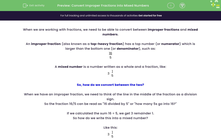# Convert Improper Fractions into Mixed Numbers

In this worksheet, students will practise converting improper or top-heavy fractions into mixed numbers (whole numbers with a fraction) by dividing by the denominator and using the remainder as the numerator in the resulting fraction.Key stage:  KS 4

Year:  GCSE

GCSE Subjects:   Maths

GCSE Boards:   Pearson Edexcel, OCR, Eduqas, AQA,

Curriculum topic:   Number, Fractions, Decimals and Percentages

Curriculum subtopic:   Structure and Calculation Fractions

Popular topics:   Numbers worksheets

Difficulty level:#### Worksheet Overview

When we are working with fractions, we need to be able to convert between improper fractions and mixed numbers.

An improper fraction (also known as a top-heavy fraction) has a top number (or numerator) which is larger than the bottom one (or denominator), such as:

 16 5

A mixed number is a number written as a whole and a fraction, like:

3
 1 5

So, how do we convert between the two?

When we have an improper fraction, we need to think of the line in the middle of the fraction as a division sign.

So the fraction 16/5 can be read as "16 divided by 5" or "how many 5s go into 16?"

If we calculated the sum 16 ÷ 5, we get 3 remainder 1.

So how do we write this into a mixed number?

Like this:

3
 1 5

We find the whole number by writing how many 5s go into 16, and the fraction by using the remainder.

This rule is ALWAYS true and can be followed in all conversions between improper fractions and mixed numbers.

In this activity, you will convert improper or top-heavy fractions into mixed numbers (whole numbers with a fraction) by dividing by the denominator and using the remainder as the numerator in the resulting fraction.

### What is EdPlace?

We're your National Curriculum aligned online education content provider helping each child succeed in English, maths and science from year 1 to GCSE. With an EdPlace account you’ll be able to track and measure progress, helping each child achieve their best. We build confidence and attainment by personalising each child’s learning at a level that suits them.

Get started